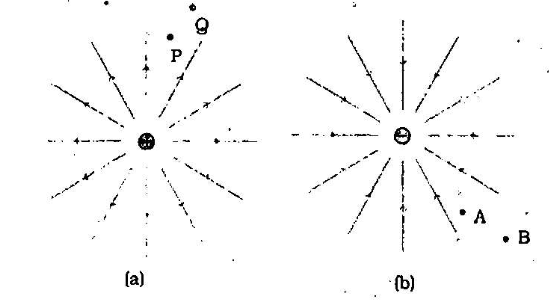Home/Class 12/Physics/

## QuestionPhysicsClass 12

Figures $$(a)$$ and $$(b)$$ show the field lines of a positive and negative point charge respectively.Give the sign of the work done by the field in moving a small positive charge from $$Q$$  to $$P$$ .

In moving a small negative charge from $$\text B$$  to $$\text A$$  work has to be done by the external agency. It is positive.